# How to Find the nth Smallest Value

This post explains that how to get the nth smallest value in a single column, or single row or an array list in excel. How to extract relative value in the same row based on the position of the nth smallest value in a single column.

## Find the nth Smallest value

You can use the SMALL function to get the 1st, 2nd, 3rd, or nth smallest value in an array or range. Also you can use the SMALL function within the INDEX function to extract the relative value of the same row. Let’s see the following formulas:

Get the First Smallest Value in the Range C2:C5

`=SMALL(C2:C5,1)`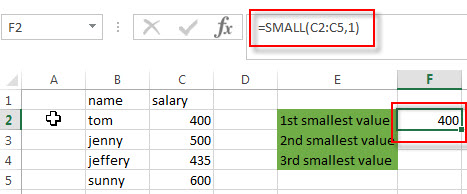This formula returns the smallest values in the range C2:C5.

Get the Second Smallest Value in the Range C2:C5

`=SMALL(C2:C5,2)`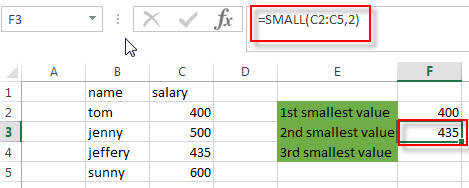The second argument of the SMALL function is set to 2, so it will extract the second smallest values in the range C2:C5.

Get the Third Smallest Value in the Range C2:C5

`=SMALL(C2:C5,3)`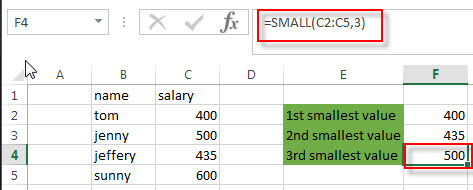To get the third smallest value in the range C2:C5, you can use the above formula.

Get the Nth Smallest Value in the Range C2:C5

So if you want to get the nth smallest value in one range, you just need to modify the second argument of the SMALL function to a numeric value that you need.

`=SMALL(C2:C5, nth)`

You can also use the SMALL function in combination with the INDEX function and MATCH function to extract the relative value in the same row position of the nth smallest in a range. For example, to get the name value in the same relative row position of the 2nd smallest salary value in the range C2:c5, you can write down the following excel formula:

`=INDEX(B2:B5,MATCH(SMALL(C2:C5,2),C2:C5,0))`

Let’s see how this formula works:

=SMALL(C2:C5,2)

This formula returns the second smallest value in the range C2:C5. It returns a numeric value.

=MATCH(SMALL(C2:C5,2),C2:C5,0)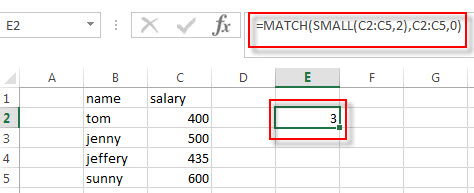The MATCH function returns the position number of the second smallest value in the range C2:C5. it returns 3.

=INDEX(B2:B5,MATCH(SMALL(C2:C5,2),C2:C5,0))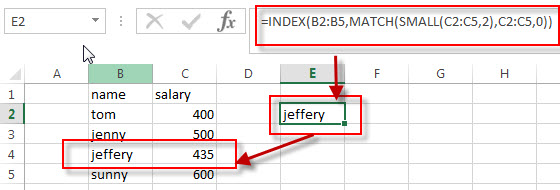The INDEX function extract the value based on the position number that returned by the above MATCH function, so it returns one value in the range B2:B5 based on the returned position. it returns “jeffery”.

### Related Formulas

• Get nth Match with One Criteria using INDEX/MATCH
if you want to find the 2th occurrence of the member “jenny” in the range B2:B10 and extracts its relative bonus value in the range D2:D10, you can used the following array formula:=INDEX(D2:D10, SMALL(IF(B2:B10=”jenny”, ROW(B2:B10)-ROW(INDEX(B2:B10,1,1))+1),2))…
• Lookup the Value with Multiple Criteria
If you want to lookup the value with multiple criteria in a range, you can use a combination with the INDEX function and MATCH function to create an array formula.…
• Lookup the Next Largest Value
If you want to get the next largest value in another column, you can use a combination of the INDEX function and the MATCH function to create an excel formula…
• Find the nth Largest Value
To get the 1st, 2nd, 3rd, or nth largest value in a range (single column, or row), you can use the LARGE function…

### Related Functions

• Excel INDEX function
The Excel INDEX function returns a value from a table based on the index (row number and column number)The INDEX function is a build-in function in Microsoft Excel and it is categorized as a Lookup and Reference Function.The syntax of the INDEX function is as below:= INDEX (array, row_num,[column_num])…
• Excel MATCH function
The Excel MATCH function search a value in an array and returns the position of that item.The syntax of the MATCH function is as below:= MATCH  (lookup_value, lookup_array, [match_type])….
• Excel SMALL function
The Excel SMALL function returns the smallest numeric value from the numbers that you provided. Or returns the smallest value in the array.The syntax of the SMALL function is as below:=SMALL(array,nth) …
Related Posts

Extract matching values From Two Lists

Suppose that you are working with two lists containing few values, and you want to extract the matching values from those two lists into another separate list. You might prefer to manually extract the matching values from the two lists, ...

Extract all Partial Matches

Just assume that you have a range of data that contains a text string and you want to extract the few partial matches into another separate range of cells; then you might think that it's not a big deal; because ...

Extract Multiple Match Values into Separate Columns

If you have a few values/items in the excel sheet and you are thinking that with the aid of the “VlOOKUP” function you can look for a specific value, extract it and then put the matching item into the separate ...

Approximate Match with Multiple Criteria by INDEX & MATCH

In Excel, INDEX function and MATCH function are often used together for returning data from specific position. And MATCH function is one of Excel lookup & reference functions that can return approximate value by setting match type. Above all, through ...

How to Sum the Smallest N Values in Excel

Sometimes we may want to sum the first smallest N numbers in a range in Excel. In this article, we will show you the method of “SUM the Smallest N Numbers” by a simple formula which consist of SUMPRODUCT and ...

Sidebar